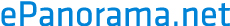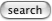# Line level signal to microphone input adapter

Sometimes there is need to convert line level signals to such signal that it can be connected to microphone input. Because the line level signals are typically in range of 0.5..2V and the microphone signals are in millivolt range, quite much attenuation is needed to match the signal levels. This means that typically you will need 40-50 dB of attenuation.

Consumer audio line (-10dBu) level to microphone matching can be done using a "40 dB L-pad attenuator". This circuit is indeed quite simple:

```+Line level input -----R1----+-- +Mic level output
|
+----R2----+
|
Ground (input)----+--------------- Ground (output)

R1 = 10 kohm
R2 = 100 ohm

```
Circuit technical data:
• Attenuation: 40 dB
• Input impedance: 10 kohm
• Output impedance: 100 ohm
• Input signal: Unbalanced line level input
• Output: Unbalanced microphone output

Professional audio audio line level (+4dBu) to microphone matching can be done using a "50 dB L-pad attenuator". This circuit is indeed quite simple:

```+Line level input -----R1----+-- +Mic level output
|
+----R2----+
|
Ground (input)----+--------------- Ground (output)

R1 = 33 kohm
R2 = 100 ohm

```
Circuit technical data:
• Attenuation: 50 dB
• Input impedance: 33 kohm
• Output impedance: 100 ohm
• Input signal: Unbalanced line level input
• Output: Unbalanced microphone output

## 20 dB PAD for line to electret microphone input

This circuit is designed to interface consumer (-10 dBu) signals to microphone input that is designed for two-were electret microphone capsules. This circuit provides around 20 dB of signal attenuation (typically enough to make thigns work, if more attenuation is needed use higher R1 value) and blocks the DC bias used in the electret microphone input to get to the line signal side (C1 does this). This circuit look like around 1 kohm signal source to at the mic side, whic matches pretty well to electret capsule characteristics (similar impedance and provides DC path for bias to go to ground).

```                 C1
+Line level in --||----R1----+-- +Mic level output
+         |
|
+----R2----+
|
Ground (input)----+--------------- Ground (output)

R2 = 1 kohm
R1 = 10 kohm
C1 = 10 uF
```
NOTE: The polarity of C1 is marked to the circuit in case you use an electrolytic capacitor. A "dry" plastic or ceramic capacitor is preferred in this circuit.

## Other attenuation values

```+Line level input -----R1----+-- +Mic level output
|
+----R2----+
|
Ground (input)----+--------------- Ground (output)
```

The attenuation of the circuit is determined by equation:

attenuation (in dB) = 20 * log10 ( (R1 + R2) / R2 )

This equation is quite accurate when the impedance of the mic input where this circuit is connected has much higher impedance than the resistance of R2. Typical microphone inputs in equipments have input impedance of 1.5 kohm or higher (which is much higher than 100 ohms).

Component selection tips:

• R2 should prefeerably be something between 10 ohms and one kilo-ohms
• R1 should be from few kilo-ohms to few hindred kilo-ohms..

Those value ranges generally works bets for audio applications.

## Constructing the circuit

The circuit itself can be built to a small metal box or even can be fitted inside an audio connector. The resistors in this circuit do not need more than 1/4W power rating (even lower ratings will do). I would recommend using metal film resistors in this circuit, because they are less noisy than cheap carbon film resistors.

I don't recommend building the circuit to a plastic box because we are playing with low level microphone signals, so the interference from nearby equipments or cellular phones can be a signnificant if you build this circuit to a plastic box or without any box. If you plan to use the circuit in any serious audio system do yourself a favor and build a circuit to a good metal box.

## What to do if I get humming noise when I connect this to my system ?

Unbalanced microphone inputs of audio equipments are very sentitive to all noise in the system, especially when you connect something else than a flowating microphone to them. Usually at short distances there are no problems in usign the adapter. If you get any 50/60 Hz noise problems, put an audio isolation transformer to teihter input or output side of my circuit.

## Balanced version

Balanced Taper Pad: 50dB (for 600 ohm impedance, XLR connector)

```   XLR in               XLR out

1 ------------------ 1

2  ---R1---+---R2--- 2
|
R3
|
3  ---R1---+---R2--- 3
```
Component values:
```R1 = 300 ohms
R2 = 300 ohms
R3 = 4 ohms
```

### Equations to calculate different value attenuations

```        ---R1---+---R2---
|
-> Z1        R3      Z2 ->
|
---R1---+---R2---
```

```R1 = ((Z1 + Z2)* 0.994 + (Z1 - Z2))/4

R2 = ((Z1 + Z2)* 0.994 - (Z1 - Z2))/4

R3 = (Z1 + Z2) / attenuation
```

Example: Z1=600 ohms Z2=600 ohms attenuation=317.3 (=50 dB)
Then R1=298 ohms, R2=298 ohms, R3=3.78 ohms

Tomi Engdahl <[email protected]>